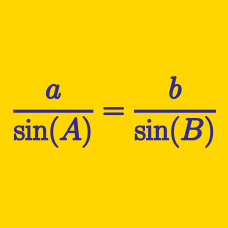Geometry

# Triangle Solving Warmup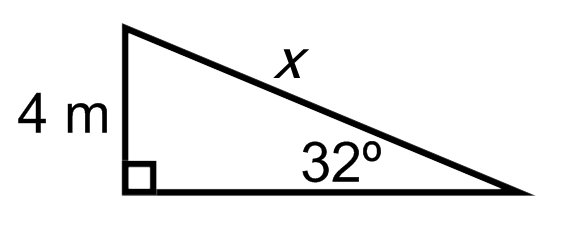What is $$x?$$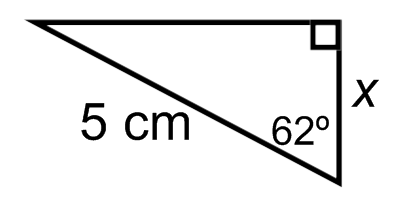What is $$x$$?Which of these is equivalent to $$\cos(a)$$?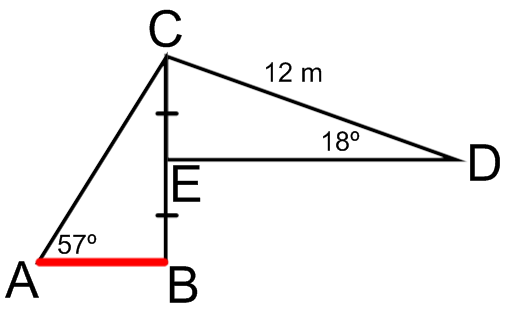What is the length of $$\overline{AB}?$$ (marked in red)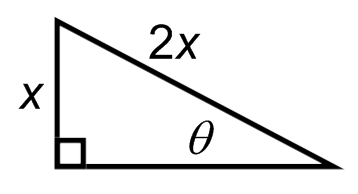What is $$\theta$$ in radians?

×

Problem Loading...

Note Loading...

Set Loading...